# Top skills in Mathematics

1 lesson

Mellan två linjer som möter varandra finns alltid en vinkel. Vi skiljer på tre olika sorters vinklar: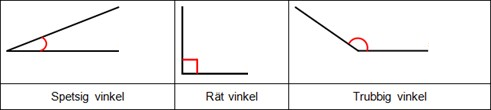Tänk på att linjernas längd inte spelar någon roll, bara hur de möter varandra. Linjerna kallas vinkelben. Den punkt de möts på kallas vinkelspets.
Vi mäter vinklar i grader. Ett helt varv är 360 grader. Ett halvt varv är 180 grader.Ett fjärdedels varv är:

Thanks to Matteboken

2 lessons

First let's see a very nice way how to practically prove that triangles always have 180° sum of angles.
Check out this video:

Cool right? You can try this yourself with any triangle you care to cut out.
Try and see if you can make one whose angles don't add up to 180.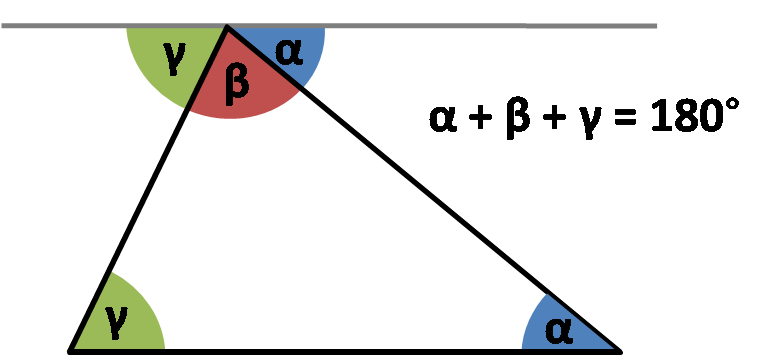Image source: Omnicalculator.com

This means that at all times we can calculate the third angle if we know the other two in any triangle.

So let's try this out!

EXERCISE:

Let's say we have a triangle just like one in the image above and let's say it's known angles are α=25°, β=75° (this doesn't necessarly have to be true for the image above, we use triangle images often more as a sketch than an actual drawing). The third angle, angle γ is unknown and we need to find it. Therefore we write:

α=25° β=75° γ=?

α + β + γ = 180°

γ = 180°- (α + β)

α + β = 25° + 75° = 100°

γ = 180°- 100°
γ = 80°

So we calculated the third angle by substracting the sum of first two from 180°.

1 lesson

## What is Fraction?

Fractions represent equal parts of a whole or a collection.

Fraction of a whole: When we divide a whole into equal parts, each part is a fraction of the whole.

For example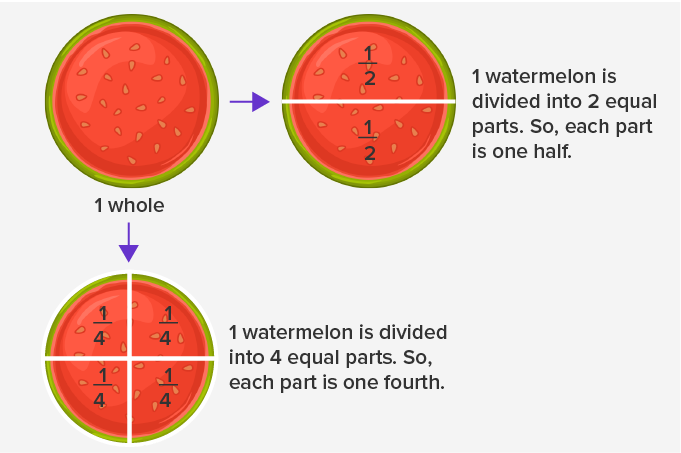Powerd by splashlearn.com
https://www.splashlearn.com/math-vocabulary/fractions/fraction

2 lessons
+1 vote
2 lessons

Estimating the weight of different objects can be successfully done if we compare them to an object of the same type, or a similar type made of the same materials.

Milk is an interesting example.
Milk is a liquid and like most everyday liquids it consists almost entirely of water - 87%, the rest is protein, milk fat, lactose, micronutrients like calcium etc. So we will estimate that milk is a little bit heavier than water. One liter of water is exactly one kilogram in weight, that is the density of water -  1 kg/l. So we can say that a liter of milk has slightly the same density (weight per kilogram), maybe a little above but close.Image source: https://www.inchcalculator.com/water-weight-calculator/

If we check it out it turns out one liter of milk is 1 kilogram and 30 grams, so for smaller amounts we can even approximate the weight of milk with a weight of water.
In fact we can approximate the weight of any liquid this way as long as it has similar density as water, we can estimate the density by examining the thickness of the substance though this is not entirely correct but for an estimation it is ok.

So a two liter bottle of juice, for example will most likely weigh around 2 kilograms as juices are almost entirely water.

+1 vote
1 lesson

A perimeter of a geometric shape would be the path we would have to travel if we would walk around the shape on its edges.
Simply speaking in order to figure out a perimeter of a geometric shape we need to know the lenght of all of its edges and sum these lengths together.

Irregular geometric shapes make it difficult as we have to know each and every edge in order to make the calculation. So if have a irregular geometric shape with four diffrent egdes : a,b,c,d... The perimetire would simply be:

P = a + b + c + d

A good example of this you can find on this link

With regular geometric shapes it is a little bit easier as they follow some rules. For example if we take rectangle, it is a geometric shape that is by definition made of four egdes, out of two and two are equal.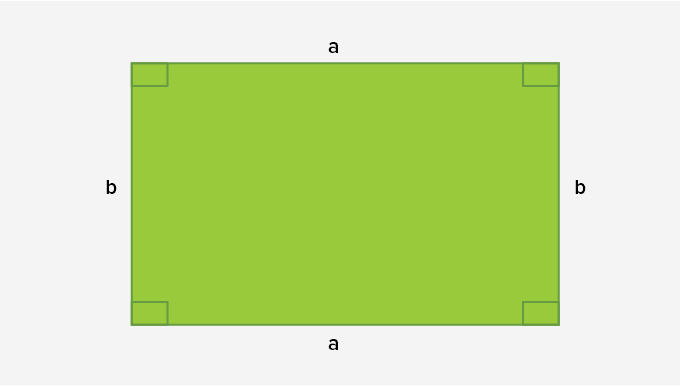Image sourced: https://www.splashlearn.com/math-vocabulary/geometry/rectangle

Taking that into account, we would write that perimeter of a rectangle is a sum of its four egdes:

P = a + a + b + b

or simpified

P = 2 x a + 2 x b

Which makes it a lot easier.

Let's try it on an examle.
Let's try and find a perimeter of some rectangles.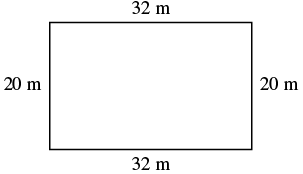Here we can se we have edge a= 32 m and edge b= 20 m (here we also use units which doesn't change things a lot).

So our perimeter should be the sum of all edges.

P = 2 x a + 2 x b
P = 2 x 32m + 2 x 20m = 64m + 40m
P = 104m

So our perimeter is 104 m and this is the procudere we will use whenever we have to calculate perimiter of a rectangle.

To find out more about calculating perimeter check out this interesting video:

+1 vote
1 lesson

The meter is the base unit from smaller units are dm, cm and mm. The prefixes deci, centi and mili that we put before meter come from latin idicating deci (like decimal) - tenth part, centi - (like century) one hudreth part and mile (as mille in italian which means thousand) a one thousandth part.

For easy conversion have in mind that we use decimal system meaning step of conversion is ten. If we are converting to smaller units we multiply with than for each step of conversion because we need to get a larger number if we count in smaller units. Just as if we would count the money in cents rather than in dollars we would get a larger number though value would be the same.Picture taken from https://www.uzinggo.com/conversion-volume-measures/proportions-measurement/math-foundations-grade-6 where you can check out for more examples

If we convert from larger to a smaller unit we would multiply with ten for each step of conversion.
The exception is when we convert from meter to kilometer we devide with a thousand but that is only beacuse in between we actually have two units that we rarely use - dekameter and hectometar.

For example if we would convert 15 m to mm we would multiply with 10 to get the value in dm (150 dm) than again with 10 to get a value in cm (1500 cm) than once more with 10 to get a value in mm (15000). So for each step of conversion we multiplied with ten and since there were three steps in between we multiplied with one thousand effectively which is exactly how many milimiters there is in one meter so if we have 15 m there is 15 000 mm in it.

+1 vote
1 lesson
all average values are integer numbers check out this video for a none integer average.

+1 vote
1 lesson

Here is a video in english that describes how to use short division

Thanks to Let's Do Math

Here is a version in swedish using staircase- and the chair method:

Thanks to Eddler

+1 vote
1 lesson

A video in english that teaches multiplaying with the box or grid method

And a video for the Lattice method

Thanks to Let's Do Math